GCSE Maths Number

Negative Numbers

# Negative Numbers

Here we will learn about negative numbers including how to add, subtract, multiply, and divide negative numbers.

There are also negative numbers worksheets based on Edexcel, AQA and OCR exam questions, along with further guidance on where to go next if you’re still stuck.

## What are negative numbers?

Negative numbers are any numbers less than zero and have a negative or minus sign () in front of them.

Numbers greater than zero are referred to as positive numbers. If there is no sign in front of a number the number is positive.

On the number line below we can see some positive and negative integers (whole numbers):

The numbers in orange are negative values and the blue numbers are positive values. Zero is neither positive or negative.

Just like you can add, subtract, multiply and divide positive numbers, you can do the same with negative numbers whether they are integers, decimals or fractions.

### What are negative numbers?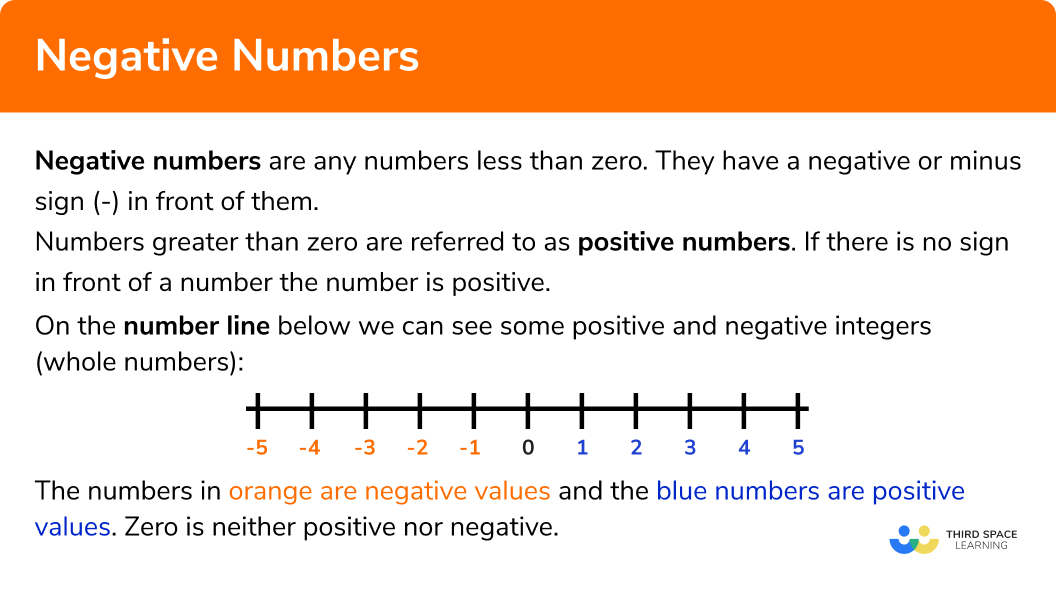### Adding and subtracting negative numbers

When adding and subtracting negative numbers use a number line:

If you are adding, move to the right of the number line.

If you are subtracting, move to the left of the number line.

Sometimes a question might have two operations next to each other:

E.g. 4 + (−2)

If the signs are the same replace with a positive sign.

If the signs are different, replace with a negative sign.

The chart below summarises this:

As a rule of thumb: same signs add, different signs subtract.

Step-by-step guide: Adding and subtracting negative numbers

### What do you need to remember when adding and subtracting negative numbers?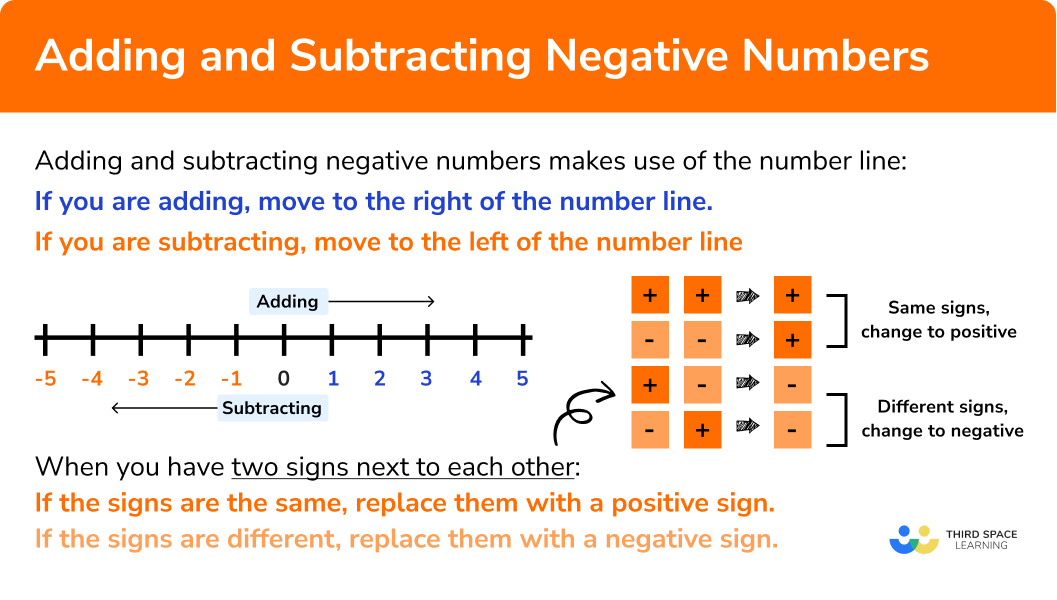### Multiplying and dividing negative numbers

Similar rules apply for multiplying and dividing negative numbers:

If the signs are the same, the answer is positive.

If the signs are different, the answer is negative.

When multiplying negative numbers:

The same rules apply for dividing negative numbers:

Step-by-step guide: Multiplying and dividing negative numbers

### What do you need to remember when multiplying and dividing negative numbers?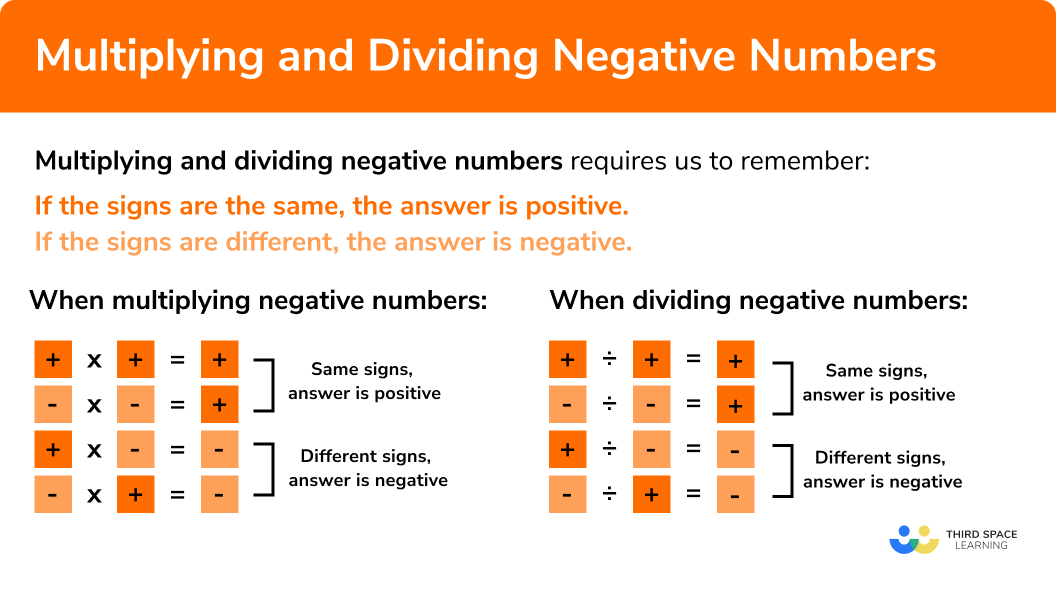## Negative number arithmetic

There are rules for calculating with negative numbers.

When adding and subtracting numbers it is important to be consistent with positive and negative values. If a number has no sign it means that it is a positive number, for example, 5 is really +5.

When two signs are written next to each other we need to remember these rules

• Two signs that are different become a negative sign
• Two signs that are the same become a positive sign

When we add and subtract negative numbers, we can use a number line to help us understand what direction we need to move.

E.g.

Work out -2-4

-2-4 = -6

Work out -4+13

-4+13=9

E.g.

Work out 4+-7

There are two different signs written next to each other. These become negative.

So,  4+-7 = 4-7

4-7= -3

When multiplying and dividing negative numbers it is often useful to complete the calculation using positive numbers initially. Then, remember the rules

• Multiplying or dividing two numbers with the same sign will give a positive answer
• Multiplying or dividing two numbers with different signs will give a negative answer

E.g.

Work out -4 ×-3

4×3 = 12

Both numbers have the same sign, so the answer will be positive.

-4 ×-3 = 12

E.g.

Work out 12 ÷-2

12÷2=6

The numbers have different signs, so the answer will be negative.

12 ÷-2 = -6

## Order integers

Integers (whole numbers) are ordered on a number line based on positive integers and negative integers. The centre of the number line is marked as 0. The integers that are greater than 0 are positive integers. The integers that are less than 0 are negative integers.

The more an integer is negative, the smaller the value is. The more an integer is positive, the bigger the value is.

E.g.

Put these numbers in ascending order.

4,-2,0, 3,-3,-5, 1, 2

Remember: ascending means smallest to biggest.

-5 is the most negative number, so this is the smallest value.

4 is the most positive number, so this is the biggest value.

So, putting them in ascending order we get, -5,-3,-2, 0, 1, 2, 3, 4

E.g.

Put these numbers in descending order.

-1, -6, -12, -4, -3, -8

Remember, descending means biggest to smallest.

-1 is the least negative number, so this is the biggest value.

-12 is the most negative number, so this is the smallest value.

So, putting them in descending order we get, -1, -3, -4, -6, -8, -12

## How to add and subtract negative numbers

In order to add and subtract negative numbers:

1. If you have two signs next to each other, change them to a single sign.
If the signs are the same, replace with a positive sign (+).
If the signs are different, replace with a negative sign ().
2. Circle the first number on the number line.
If you are adding, move to the right of the number in step 2 ().
If you are subtracting, move to the left of the number in step 2 ().

### Explain how to add and subtract negative numbers in 4 steps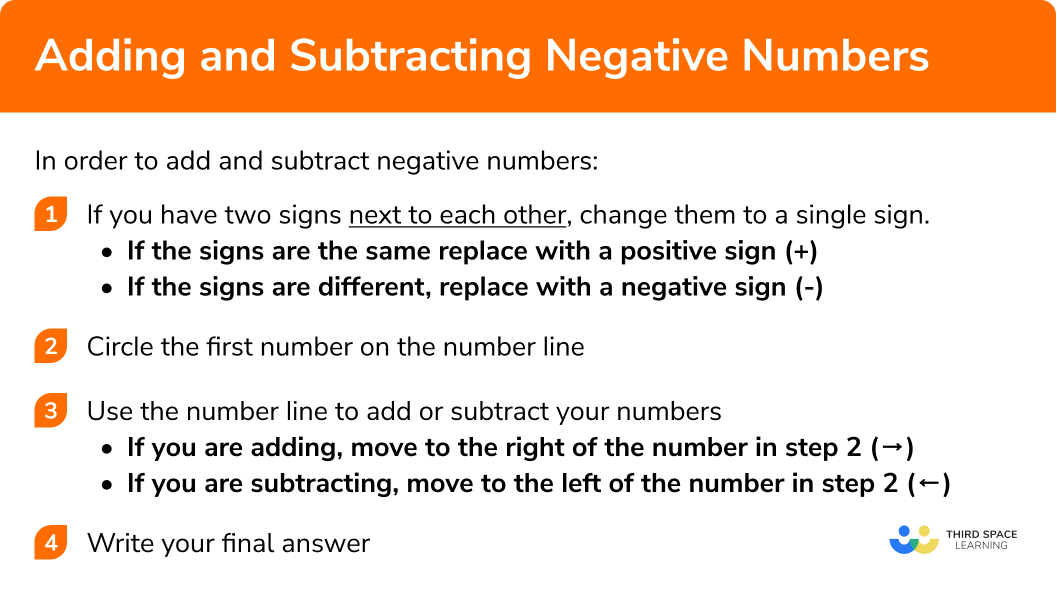## How to multiply and divide negative numbers

In order to multiply and divide negative numbers:

1. Multiply or divide the numbers normally.
2. Change the sign as necessary using the rules of multiplying and dividing negative numbers:
If the signs are the same, the answer is positive.
If the signs are different, the answer is negative.

### Explain how to multiply and divide negative numbers in 2 steps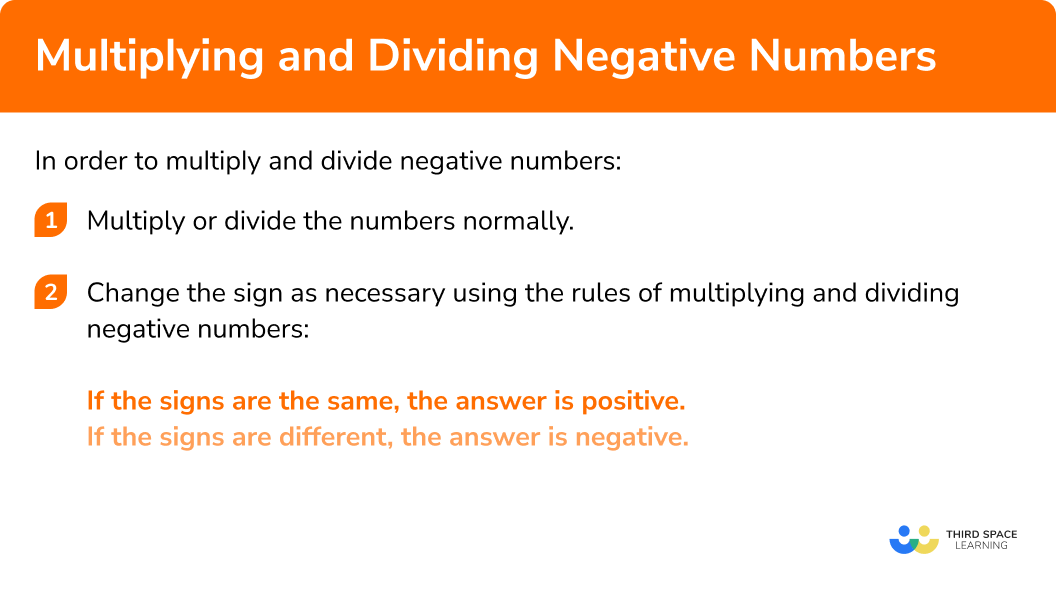## Negative numbers examples

### Example 1: adding a positive number

$−5 + 8$

1. If you have two signs next to each other, change them to a single sign.

If the signs are the same in the middle of the calculation, replace with a positive sign (+).

If the signs are different, replace with a negative sign ().

In this case you do not have two signs next to each other.

2Circle the first number on the number line.

The first number in the question is (−5)

If you are adding, move to the right of the number in step 2 (→).

If you are subtracting, move to the left of the number in step 2 (←).

In this case we are adding the 8 so move 8 spaces right from the (-5) on the number line:

$-5 + 8 = 3$

### Example 2: subtracting a negative number (two operations)

$−7 − (−5)$

If you have two signs next to each other, change them to a single sign.

Circle the first number on the number line.

### Example 3: multiplying negative numbers

$−6 × (−7)$

Multiply or divide numbers normally.

Change the sign using the rules of multiplying and dividing negative numbers:

### Example 4: dividing negative numbers

$-25 ÷ 5$

Multiply or divide numbers normally.

Change the sign using the rules of multiplying and dividing negative numbers:

### Example 5: mixed operations (Order of operations)

$-18 ÷ (-6) – 3 × (-2)^2=$

Multiply or divide the numbers normally.

Change the sign using the rules of multiplying and dividing negative numbers:

Circle the first number on the number line.

### Example 6: real life word problem

The table below shows the temperatures recorded in Leicester at different times of the day.

a) What is the product of the highest and lowest temperatures?

b) What is the difference between the temperatures at 2am and 1pm?

Time of Day

2 am

7 am

1 pm

6 pm

Temperature ( ^{\circ}C )

−8

−5

3

−1

To calculate a):

Write down the highest and lowest temperatures.

Multiply or divide numbers normally.

Change the sign using the rules of multiplying and dividing negative numbers:

To calculate b):

If you have two signs next to each other, change them to a single sign.

Circle the first number on the number line.

If you are adding, move to the right of the number in step 2 (→).
If you are subtracting, move to the left of the number in step 2 (←).

### Common misconceptions

• Greater negative does mean a larger number

Students sometimes assume that the larger a negative number the greater it is. For example, students might incorrectly assume -3 is greater than 2 because 3 is a larger number.

• Raising a negative number to a power greater than one

Remember when raising a negative number to a power greater than 1 the resulting answer could be positive or negative. When you raise a negative number to an odd exponent the resulting answer is negative, when you raise a negative number to an even exponent the resulting answer is positive.

• Changing signs when adding and subtracting
When adding or subtracting with negative numbers the signs change only if they are next to each other in the middle of the calculation and are different

### Practice negative numbers questions

1. Work out: -8+10

-22-18182. Work out: -11-(-8)

-33-1919We have – \; – together so change it to a + .

-11+8 = -3

3. Work out: -8 \times (-9)

-727217-178 \times 9 = 72

The signs are the same so the answer is positive.

-8 \times (-9)=72

4. Work out: -144 \div 3

147-147-4848-144 \div 3 = 48

The signs are different so the answer is negative.

-144 \div 3= -48

5. Calculate: 18 \div (-9) +5 \times (-3)^2=

-47-434327We need to follow BIDMAS here:

(-3)^2=-3 \times -3=9

5 \times 9=45

18 \div (-9)=-2

So we have

-2+45=43

6. The table shows the temperature in Manchester at different times of the day.

Time of the Day          Temperature   (^{\circ}C)

1am -8
4am -2
7am 2
11am -9

What is the difference between the highest and lowest temperatures?

1^{\circ}C7^{\circ}C10^{\circ}C11^{\circ}CThe calculation we need to do here is 2 – – 9. There is a – \; – together so this becomes a +.

2–9 = 2 + 9 = 11

### Negative numbers GCSE questions

1.   Tilly has the following 6 cards:She is going to choose 3 cards and multiply them.

(a)   What is the largest possible number she can make?

(b)   What is the smallest possible number she can make?

(6 Marks)

(a)

For identifying 2 of the following: -9 or -8 or 7 .

(1)

Multiply -9, and -8 to get 72 .

(1)

Correctly multiplying -9, -8 and 7 or 504 seen.

(1)

(b)

For identifying 2 of the following: -9 or 7 or 3 .

(1)

Shows working how to multiply.

(1)

Correctly multiplying -9, 7 and 3 or -189 seen.

(1)

2.  Mr. and Mrs. Brown had \pounds 198.78 in their bank account. At the end of the month they had to pay 4 bills

They paid the tv license of \pounds 57.20 , utilities of \pounds 134.78 , car insurance \pounds 38.25 , and credit card bill of \pounds 94.

How much were the Browns overdrawn?

(3 Marks)

Finding the sum of the 4 bills.

(1)

Subtracting the cost of the four bills from the balance in the bank account.

(1)

cao

-\pounds 125.45

(1)

3.  Below is a list of cities and their elevations:

City                         Elevation

Helsinki                  25m

New Orleans          -2m

Buenos Aires          10m

Amsterdam              -7m

Baku                        -28m

(a)   Which cities are below sea level?

(b)   What is the difference between the elevation of Buenos Aires and Baku?

(5 Marks)

(a)

Baku

(1)

Amsterdam

(1)

New Orleans

(1)

(b)

10 – (-28)

(1)

38m

(1)

## Learning checklist

You have now learned how to:

• Add and subtract integers both positive and negative
• Multiply and divide integers both positive and negative
• Use negative numbers in context and calculate intervals across 0

## Still stuck?

Prepare your KS4 students for maths GCSEs success with Third Space Learning. Weekly online one to one GCSE maths revision lessons delivered by expert maths tutors.

Find out more about our GCSE maths tuition programme.